## LetsPlayMaths.Com

WELCOME TO THE WORLD OF MATHEMATICS

# Class 4 Factors and Multiples

Divisibility

Even Numbers

Odd Numbers

Factors of a Number

Prime Numbers

Composite Numbers

Multiples

Factors / Multiple Test

Factors / Multiple Worksheet

Answer Sheet

## Divisibility

When a number is divided by another number and the remainder becomes zero, then we say the larger number is divisible by the smaller number. There are various rules to find out whether a number is divisible by another number. Few rules are given below.

Divisible by 2

A number is divisible by 2 if it’s last digit is having 0 / 2 / 4 / 6 / 8.

Example 1. Check if 12436 is divisible 2.

Solution. As per the rule 12436 should be divisible by 2 as it’s last digit is 6. Let’s verify it.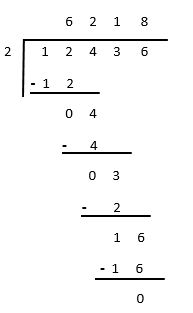Hence, it is proved that 12436 is divisible by 2.

Example 2. Check if 10345 is divisible by 2.

Solution. As per the rule 10345 should not be divisible by 2 as it’s last digit is 5. Let’s verify it.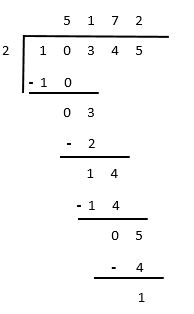Remainder is 1, hence it is proved that 10345 is not divisible by 2.

Divisible by 3
A number is divisible by 3 when the sum of it’s digits is divisible by 3.

Example 1. Check if 10245 is divisible by 3.

Solution. Addition of all the digits of 10245 = 1 + 0 + 2 + 4 + 5 = 12
12 is divisible by 3.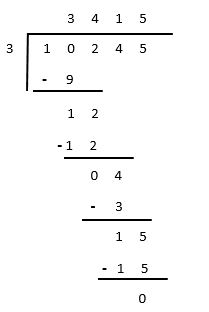Remainder is zero, so it is proved that 10245 is divisible by 3.

Example 2. Check if 25435 is divisible by 3.

Solution. Addition of all the digits of 25435 = 2 + 5 + 4 + 3 + 5 = 19
19 is not divisible by 3, that means 25435 is not divisible by 3. Let’s prove it.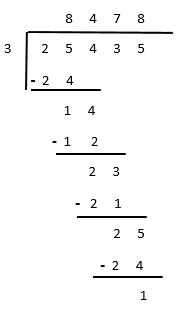Remainder is 1, so it is proved that 25435 is not divisible by 3.

Divisible by 4

A number is divisible by 4 if the number formed by it’s last two digits is divisible by 4.

Example 1. Check if 32064 is divisible by 4.

Solution. Last two digits of 32064 is 64 and it is divisible by 4. That means 32064 is divisible by 4.
Let’s verify it.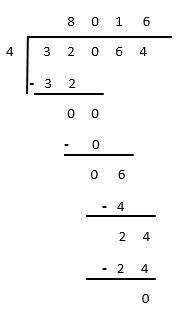Remainder is 0, that means 32064 is divisible by 4.

Example 2. Check if 25218 is divisible by 4.

Solution. Last two digit of 25218 is 18, but it is not divisible by 4. Let’s check it.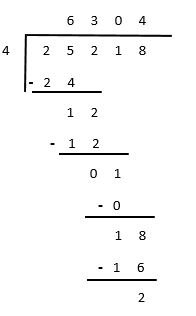Remainder is 2, so it is proved that 25218 is not divisible by 4.

Divisible by 5

A number is divisible by 5 if it’s last digit is 0 or 5.

Example 1. Check if 2765 is divisible by 5.

Solution. Last digit of 2765 is 5, so it is divisible by 5. Let’s verify it by doing the division.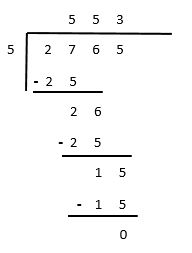Remainder is zero, so it is proved that 2765 is divisible by 5.

Example 2. Check if 4820 is divisible by 5.

Solution. Last digit of 4820 is 0, so it is divisible by 5. Let’s verify it by doing the division.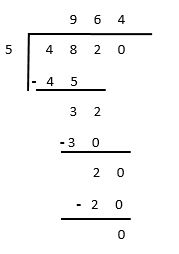Remainder is zero, so it is proved that 4820 is divisible by 5.

Example 3. Check if 6524 is divisible by 5.

Solution. Last digit of 6524 is 4, so it is not divisible by 5. Let’s verify it by doing the division.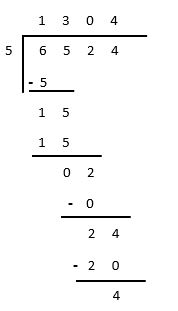Remainder is 4, so it is proved that 6524 is not divisible by 5.

Divisible by 6

A number is divisible by 6, if it is divisible by both 2 and 3. We first check if it is divisible by 2 and then by 3.

Example 1. Check if 21036 is divisible by 6.

Solution. Last digit of 21036 is 6, so it is divisible by 2.
Addition of all the digits of 21036 = 2 + 1 + 0 + 3 + 6 = 12
12 is divisible by 3, so 21036 is divisible by 3. Now we can say that 21036 is divisible by 6, as it is divisible
by both 2 and 3. Let’s verify it by dividing 21036 by 6.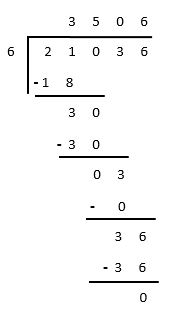Remainder is 0, so it is proved that 21036 is divisible by 6.

Example 2. Check if 5234 is divisible by 6.

Solution. Last digit of 5234 is 4, that means it is divisible by 2.
Addition of all the digits present in 5234 = 5 + 2 + 3 + 4 = 14
14 is not divisible by 3. As per the rule, a number can be divisible by 6 if it is divisible by both 2 and 3. 5234
is divisible by 2, but it is not divisible by 3. So, it is not divisible by 6. Let’s verify it by dividing 5234 by 6.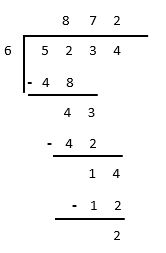Remainder is 2, so 5234 is not divisible by 6.

Divisible by 8

A number is divisible by 8 if the number formed by it’s last three digits is divisible by 8.

Example 1. Check if 25248 is divisible by 8.

Solution. The number formed by last three digits of 25248 is 248 and it is divisible by 8.
248 ÷ 8 = 31
Let’s verify it by dividing 25248 by 8.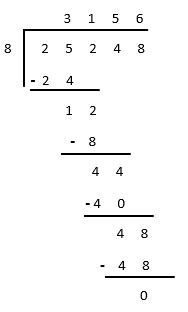Remainder is 0, so 25248 is divisible by 8.

Divisible by 9

A number is divisible by 9 if the sum of it’s digits is divisible by 9.

Example 1. Check if 5922 is divisible by 9.

Solution. Addition of all the digits of 5922 = 5 + 9 + 2 + 2 = 18
18 is divisible by 9, as per the rule 5922 should be divisible by 9. Let’s verify it by dividing 5922 by 9.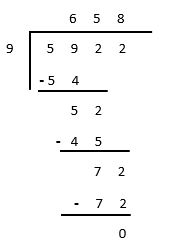Remainder is 0, so it is proved that 5922 is divisible by 9.

Example 2. Check if 4729 is divisible by 9.

Solution. Addition of all the digits of 4729 = 4 + 7 + 2 + 9 = 22
22 is not divisible by 9. So, as per the rule 4792 is not divisible by 9. Let’s verify it by dividing 4792 by 9.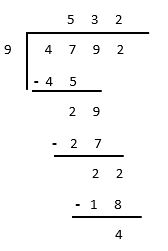Remainder is 4, so it is proved that 4792 is not divisible by 9.

## Even Numbers

Number that is divisible by 2 is known as Even Number.All the even numbers last digit will always
have 0 / 2 / 4 /6 / 8.

Example 1. Check if 2586 is an even number.

Solution. Last digit of 2586 is 6 and it is divisible by 2. Since 2586 is divisible by 2, so it is an even number.

Example 2. Check if 573 is an even number.

Solution. Last digit of 573 is 3, and it is not divisible by 2. So, 573 is not an even number.

## Odd Numbers

Number that is not divisible by 2 is known as Odd Number.

Example 1. Check if 235 is an odd number.

Solution. Last digit of 235 is 5 and it is not divisible by 2. So, 235 is an odd number.

Example 2. Check if 1578 is an odd number.

Solution. last digit of 1578 is 8 and it is divisible by 2. Since 1578 is divisible by 2, hence it is an odd number.

## Factors of a Number

A number is said to be a factor of another number, if it divides the other number completely. For example, 16 is completely divisible by 2, so we can say that 2 is a factor of 16.

Factors of 12
We must find out by how many numbers 12 is completely divisible. Or, we must find out the different multiplication
factors of 12.

1 X 12 = 12
2 X 6 = 12
3 X 4 = 12
So, factors of 12 are 1, 2, 3, 4, 6 & 12.

Factors of 16

1 X 16 = 16
2 X 8 = 16
4 X 4 = 16
So, factors of 16 are 1, 2, 4 & 16

## Prime Numbers

Number which are divisible by 1 and itself are known as Prime Numbers. In other words, prime numbers have only two factors, that is 1 and number itself. Few examples of prime numbers are given below.

2, 3, 5, 7, 11, 13, 17, 19, 23, 29, 31, etc.

## Composite Numbers

Numbers that have more than two factors is known as Composite Numbers.Few examples are given below.

4, 6, 8, 9, 10, 12, 14, 15, 16, 18, 20, 21, 22, 24, 25, 26, 27, 28, 30, 32, etc.

## Multiples

Multiple of a number is any of the products of the given number and some other counting number.
For example, lets see the multiples of 3.

 3 X 1 3 3 X 2 6 3 X 3 9 3 X 4 12 3 X 5 15 3 X 6 18 3 X 7 21 3 X 8 24 3 X 9 27

## Factors / Multiple Test

Factors & Multiples Test - 1

Factors & Multiples Test - 2

## Class-4 Factors and Multiples Worksheet

Factors & Multiples Worksheet - 1

Factors & Multiples Worksheet - 2

## Answer sheet

Factors-Multiples-AnswerDownload the pdf

Copyright © 2021 LetsPlayMaths.com. All Rights Reserved.